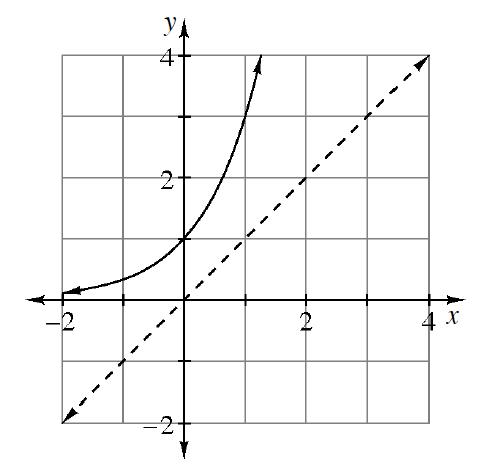### Home > PC3 > Chapter 1 > Lesson 1.2.3 > Problem1-97

1-97.The graph of an exponential function is sketched below. Sketch the graph of the inverse and state the domain and range for the function and its inverse. The dotted line is $y=x$

Since the point $(0,1)$ is on the original function, the point $(1,0)$ is on the inverse function.